# Java program to convert uppercase to lowercase

This article is created to cover a program in Java that converts an uppercase character or string to its equivalent lowercase version with or without the use of a string function. Here is the list of programs covered in this article:

• Convert uppercase characters to lowercase using ASCII (Basic Version)
• Convert uppercase characters to lowercase using ASCII (complete version)
• Convert an uppercase string to lowercase without using the string function
• Convert uppercase string to lowercase using the string function

The string function I'm talking about is toLowerCase().

Note: ASCII values for A-Z are 65–90. And the ASCII values of a–z are 97–122.

Note: The ASCII value of 'a' is 97, whereas the ASCII value of 'A' is 65. The ASCII value of a lowercase character to its equivalent uppercase is 32 more. Therefore, adding 32 to the ASCII value of an uppercase character becomes the ASCII value of its equivalent lowercase character.

## Uppercase character to lowercase in Java using ASCII

The question is: write a Java program to convert an uppercase character to its equivalent lowercase character using the ASCII value of the character. The character must be received by the user at the runtime of the program. The program given below has its answer:

```import java.util.Scanner;

public class CodesCracker
{
public static void main(String[] args)
{
char chUpper, chLower;
int ascii;
Scanner scan = new Scanner(System.in);

System.out.print("Enter a Character in Uppercase: ");
chUpper = scan.next().charAt(0);

ascii = chUpper;
ascii = ascii + 32;
chLower = (char)ascii;

System.out.println("\nEquivalent Character in Lowercase = " +chLower);
}
}```

The snapshot given below shows the sample output produced by the above Java program. This is the initial or first output: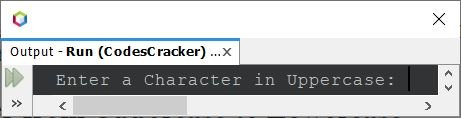Now enter a character in uppercase, say Y, and press the ENTER key to convert and print the same character back on the output screen, but in lowercase, as shown in the snapshot given below: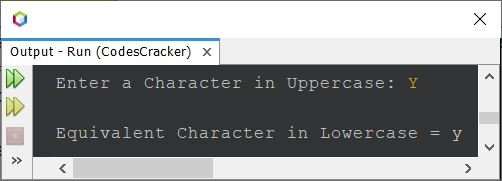The main block of code in the above program is the following:

```ascii = chUpper;
ascii = ascii + 32;
chLower = (char)ascii;```

Using the first statement, the ASCII value of the character stored in the variable chUpper gets initialized to ascii. This is because chUpper is of char type, whereas ascii is of int. We need not cast while converting from lower to higher data types because of the type promotion rules. But in the third statement, we need to cast from int to char, or ASCII to its equivalent character.

## Uppercase Character to Lowercase in Java: Complete Version

Since the previous program produced the wrong output, when the user enters a character already in lowercase or any character other than the alphabet, therefore, I've modified that program. Here is the modified version of the previous program:

```import java.util.Scanner;

public class CodesCracker
{
public static void main(String[] args)
{
Scanner scan = new Scanner(System.in);

System.out.print("Enter an Alphabet in Uppercase: ");
char chUpper = scan.next().charAt(0);

int ascii = chUpper;
if(ascii>=65 && ascii<=90)
{
ascii = ascii + 32;
char chLower = (char)ascii;
System.out.println("\nEquivalent Character in Lowercase = " +chLower);
}
else if(ascii>=97 && ascii<=122)
else
System.out.println("\nInvalid Input!");
}
}```

The snapshot given below shows a sample run of the above Java program with user input 2.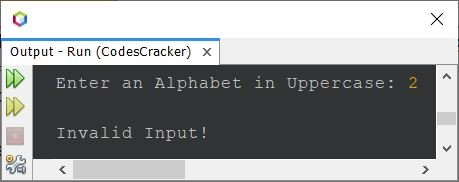Here is another sample run with user input, r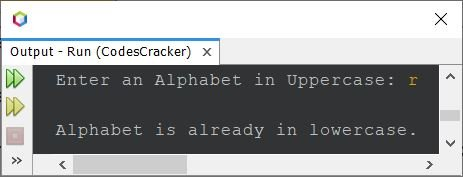## Uppercase String to Lowercase in Java without using the String Function

This program converts a string from uppercase to lowercase without using the string function. I've used ASCII values to do the job in a similar way while doing the conversion of uppercase characters to lowercase, as in the previous program.

```import java.util.Scanner;

public class CodesCracker
{
public static void main(String[] args)
{
String strUpper;
int i, ascii;
char ch;
Scanner scan = new Scanner(System.in);

System.out.print("Enter the String: ");
strUpper = scan.nextLine();

int len = strUpper.length();
char[] strUpperChars = new char[len];
char[] strLowerChars = new char[len];
for(i=0; i<len; i++)
strUpperChars[i] = strUpper.charAt(i);

for(i=0; i<len; i++)
{
ch = strUpperChars[i];
ascii = ch;
if(ascii>=65 && ascii<=90)
{
ascii = ascii + 32;
ch = (char)ascii;
strLowerChars[i] = ch;
}
else
strLowerChars[i] = ch;
}

System.out.print("\nString in Lowercase: ");
for(i=0; i<len; i++)
System.out.print(strLowerChars[i]);
}
}```

## Convert a string from uppercase to lowercase in Java using the String Function

This is the last program in this article, created using a string function named toLowerCase() that converts the whole string into lowercase.

```import java.util.Scanner;

public class CodesCracker
{
public static void main(String[] args)
{
Scanner scan = new Scanner(System.in);

System.out.print("Enter the String: ");
String strUpper = scan.nextLine();

String strLower = strUpper.toLowerCase();
System.out.println("\nEquivalent String in Lowercase: " +strLower);
}
}```

The snapshot given below shows the sample run of the above Java program with user input cODEsCRacker @fO45 as a string to convert and print its equivalent string in lowercase: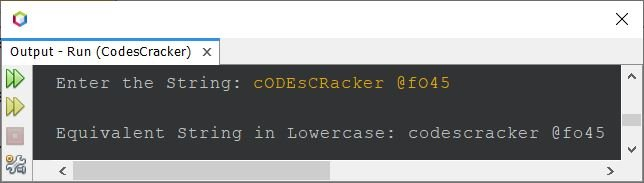See how the short program becomes after using a library function named toLowerCase(). To make it shorter, the same program can also be written as:

```import java.util.Scanner;

public class CodesCracker
{
public static void main(String[] args)
{
Scanner scan = new Scanner(System.in);
System.out.print("Enter the String: ");
String str = scan.nextLine();
System.out.println("\nEquivalent in Lowercase: " +str.toLowerCase());
}
}```

You'll get the same output as the previous program.

#### Same Program in Other Languages

Java Online Test

« Previous Program Next Program »